MPM 2 D Quadratic Word Problems Optimization Problems

• Slides: 12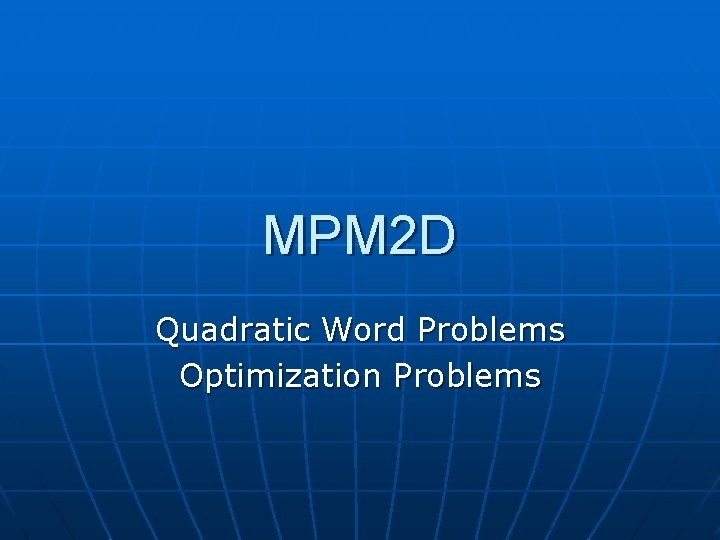MPM 2 D Quadratic Word Problems Optimization Problems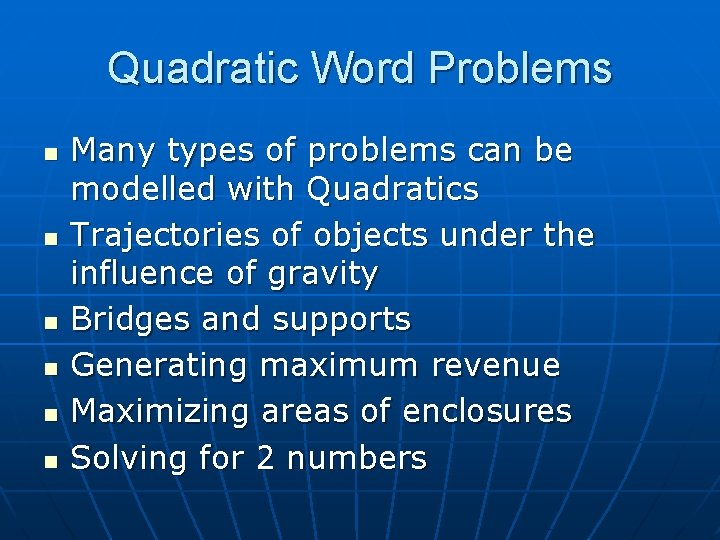Quadratic Word Problems n n n Many types of problems can be modelled with Quadratics Trajectories of objects under the influence of gravity Bridges and supports Generating maximum revenue Maximizing areas of enclosures Solving for 2 numbersQuadratic Word Problems n n n Each problem will require you to assign algebraic letters to things you solve for Many times it will be a letter for each of the dependent and independent variables Choose a letter that makes sense: R for revenue, P for profit, A for area, etc Make “Let” statements: Let “x” represent one number and “w” (or whatever) the other number. (Don’t use y as we need it for the equations later. ) Reword the question in your own words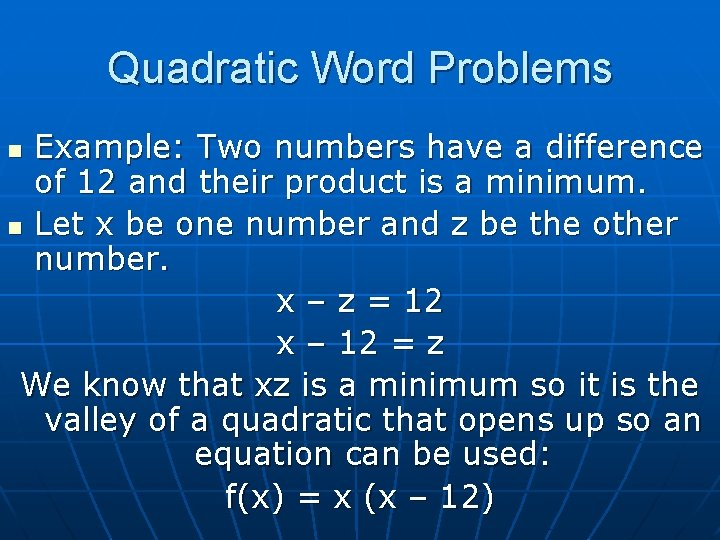Quadratic Word Problems Example: Two numbers have a difference of 12 and their product is a minimum. n Let x be one number and z be the other number. x – z = 12 x – 12 = z We know that xz is a minimum so it is the valley of a quadratic that opens up so an equation can be used: f(x) = x (x – 12) nQuadratic Word Problems f(x) = x (x – 12) = 0 Use the zeroes: x = 0, x = 12 The vertex x is (0+12)/2 x=6 f(6) = 6(6 – 12) = - 36 The vertex is: (6, – 36) So x = 6 is one of the numbers. z = x – 12 = 6 – 12 = – 6 The two numbers are 6 and – 6.Another question n Find two numbers whose sum is 48 and whose product is a maximum.Revenue n n n With Revenue: you let R be the revenue and the number of increases in the cost of the item be x or whatever. Usually the question starts with the cost of an item selling a certain number and when the cost is raised, fewer items are sold. The maximum (peak of the quadratic) shows when max revenue is generated (when the number of items and their cost yields the best revenue!!)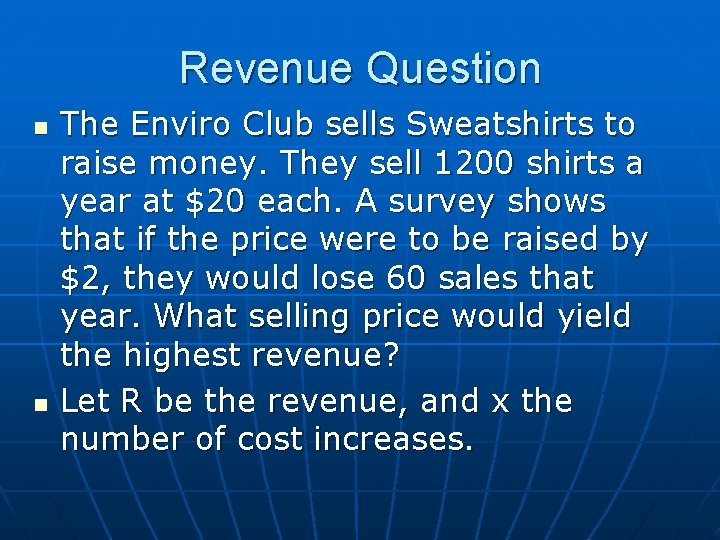Revenue Question n n The Enviro Club sells Sweatshirts to raise money. They sell 1200 shirts a year at \$20 each. A survey shows that if the price were to be raised by \$2, they would lose 60 sales that year. What selling price would yield the highest revenue? Let R be the revenue, and x the number of cost increases.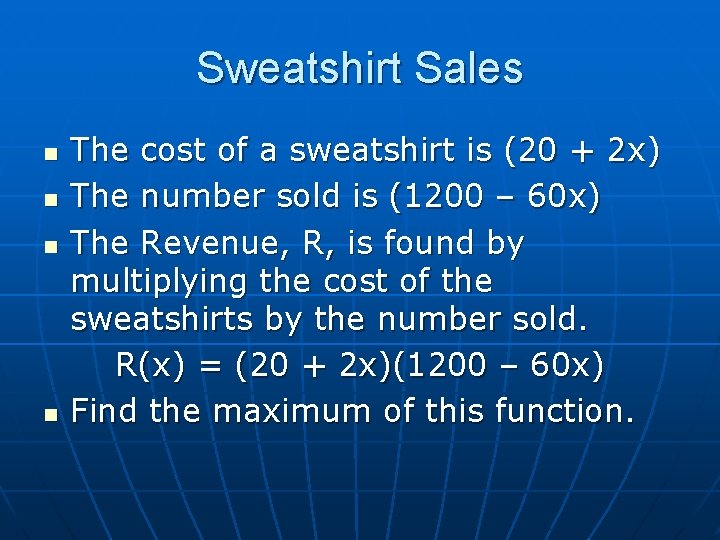Sweatshirt Sales n n The cost of a sweatshirt is (20 + 2 x) The number sold is (1200 – 60 x) The Revenue, R, is found by multiplying the cost of the sweatshirts by the number sold. R(x) = (20 + 2 x)(1200 – 60 x) Find the maximum of this function.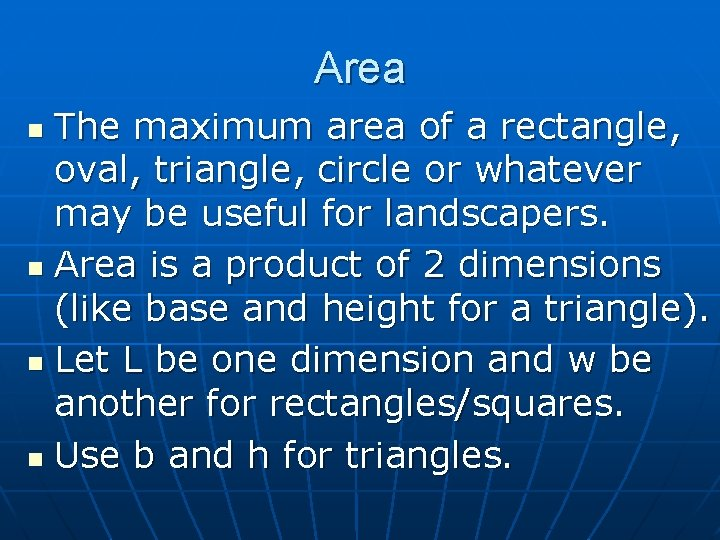Area The maximum area of a rectangle, oval, triangle, circle or whatever may be useful for landscapers. n Area is a product of 2 dimensions (like base and height for a triangle). n Let L be one dimension and w be another for rectangles/squares. n Use b and h for triangles. nArea Question n n Determine the maximum area of a triangle in square centimetres. The sum of its base and height must be 10 cm. Let b be the base and h be the height (naturally!) h + b = 10 h = 10 – b Area = bh/2 A = 0. 5(b)(10 – b)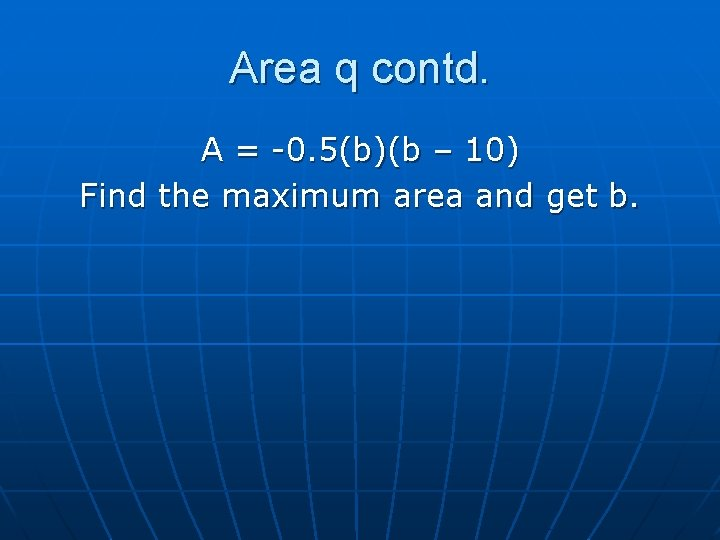Area q contd. A = -0. 5(b)(b – 10) Find the maximum area and get b.